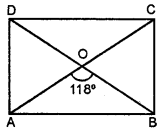## ML Aggarwal Class 8 Solutions for ICSE Maths Chapter 13 Understanding Quadrilaterals Ex 13.3

Question 1.
Identify all the quadrilaterals that have
(i) four sides of equal length
(ii) four right angles.
Solution:
(i) Any quadrilateral whose four sides are equal
in length is a square or rhombus.
(ii) A quadrilateral having four right angles
is a square or a rectangle.

Question 2.
Explain how a square is
(ii) a parallelogram
(iii) a rhombus
(iv) a rectangle.
Solution:
(i) A square is a quadrilateral which has four sides
and four angles whose sum is 360°.
(ii) A square is a parallelogram whose opposite sides are parallel.
(iii) A square is a parallelogram whose sides are equal
and so, it is a rhombus.
(iv) A square is a parallelogram whose each angle is 90°.
So, it is a rectangle.

Question 3.
(i) bisect each other
(ii) are perpendicular bisectors of each other
(iii) are equal.
Solution:
(i) Rectangle, square, rhombus, parallelogram.
(ii) Square, rhombus.
(iii) Square, rectangle.

Question 4.
One of the diagonals of a rhombus and its sides are equal. Find the angles of the rhombus.
Solution:
In a rhombus, side and one diagonal are equal.
∴ Angles will be 60° and 120°.Question 5.
In the given figure, ABCD is a rhombus, find the values of x, y and z.Solution:
In rhombus ABCD.
∵ The diagonals of rhombus bisect each other at right angles.
∴ AO = OC and BO = OD
AO = x, OC = 8 cm, BO =y and OD = 6 cm
∴ x = 8 cm and y = 6 cmIn ∆AOB,
AB2 = AO2 + BO2
AB2 = 82 + 62
AB2 = 64 + 36
AB2 = 100 = (10)2
AB = 10 cm

Question 6.
In the given figure, ABCD is a trapezium. If ∠A : ∠D = 5 : 7, ∠B = (3x + 11)° and ZC = (5x – 31)°, then find all the angles of the trapezium.Solution:
In the given figure,
ABCD is a trapezium in which DC || AB
∠A : ∠D = 5 : 7
∠B = (3x + 11)° and
∠C = (5x – 31)°
∵ ABCD is a trapezium
∴ ∠B + ∠C = 180° (Cointerior angle)
3x + 11° + 5x – 31° = 180°
8x – 20° = 180° ⇒ 8x = 180° + 20° = 200°
⇒ x = $$x=\frac{200^{\circ}}{8}=25^{\circ}$$
2x = 180°- 118°
2x = 62° ⇒ x = 31°
∴ ∠ABO = 31°

(ii) Also, ∠AOB + ∠AOD = 180° (Linear pair)
∴ ∠AOD = 180°- 118° = 62°
Now, In ∆AOD, AO = DO = y°
∴ 62 + 2y= 180°
2y = 180° – 62°
2y = 180°- 62°
2y = 118° ⇒ y = 59°

(iii) ∠OCB = ∠OAD = 59° (Alternate angles)

Question 7.
In the given figure, ABCD is a rectangle. If ∠CEB : ∠ECB = 3 : 2 find
(i) ∠CEB,
(ii) ∠DCFSolution:
In ∆ BCE, ∠B = 90° (∵ ABCD is a rectangle)
∴ ∠CEB + ∠ECB = 90°
3x + 2x = 90°
⇒ x= 18°
∴ ∠CEB = 3x = 3 × 18° = 54°
Now, ∠CEB = ∠ECD = 54° (Alternate angles)
Also ∠ECD + ∠DCF = 180° (Linear pair)
⇒ ∠DCF = 180 – 54= 126°

Question 8.
In the given figure, ABCD is a rectangle and diagonals intersect at O. If ∠AOB = 118°, find(i) ∠ABO
(iii) ∠OCB
Solution:
In ∆ ABO, OA = OB
(∵ diagonals of a rectangle bisect each other)∴ ∠OAB = ∠OBA = x °
⇒ 118° + x + x = 180°
2x= 180°- 118°
2x = 62° ⇒ x = 31°
∴ ∠ABO = 31°

(ii) Also, ∠AOB + ∠AOD = 180° (Linear pair)
∴ ∠AOD = 180° – 118° = 62°
Now, In ∆ AOD, AO = DO = y°
∴ 62 + 2y = 180°
2y = 180°- 62°
2y = 118° ⇒ y = 59°

(iii) ∠OCB = ∠OAD = 59° (Alternate angles)

Question 9.
In the given figure, ABCD is a rhombus and ∠ABD = 50°. Find :
(i) ∠CAB
(ii) ∠BCDSolution:
(i) We know that diagonals of a rhombus
are ⊥ to each other.∴ ∠BOA = 90°
In ∆ AOB,
∠OAB + ∠BOA + ∠ABO = 180°
∠OAB + 90° + 50° = 180°
∠OAB = 180 – 140 = 40°
∴ ∠CAB = ∠OAB = 40°

(ii) ∠BCD = 2 ∠ACD = 2 × 40° = 80°
(∵ ∠CAB = ∠ACD alternate angles)
(iii) ∠ADC = 2 ∠BDC = 2 × 50° = 100°
(∵ ∠ABD = ∠BDC alternate angles)

Question 10.
In the given isosceles trapezium ABCD, ∠C = 102°. Find all the remaining angles of the trapezium.Solution:
AB || CD
∠B + ∠C = 180°
(∵ adjacent angles on the same side of
transversal are supplementary)
⇒ ∠B + 102° = 180°
∠B = 180°- 102° = 78°
∴ ∠A = ∠B = 78°
∠A + ∠B + ∠C + ∠D = 360°
78° + 78° + 102° + ∠D = 360°
∠D + 258° = 360°
∠D = 102°

Question 11.
In the given figure, PQRS is a kite. Find the values of x and y.Solution:
In the figure, PQRS is a kite
∠Q = 120° and ∠R = 50°
∴ ∠Q = ∠S
∴ x = 120°
∠P + ∠R = 360° – (120° + 120°)
∠P + ∠R = 360° – 240° = 120°
But ∠R = 50°
∴ ∠P = y = 120°- 50° = 70°
Hence, x = 120°, y = 70°